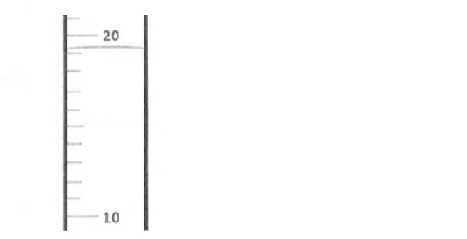# Problem: At 4.000°C water’s density is 1.000 g/cm 3. For the range of temperatures associated with “room temperature” the density of pure water is: Density (g/cm 3) = (-0.00030 (g/°C • cm 3) x Temperature (°C) + 1.0042 (g/cm 3) Based on this thermometer reading, how many significant figures should you report for the calculated density? a) One                   b) Two                  c) Three                   d) Four                 e) Five

###### FREE Expert Solution
96% (199 ratings)
###### Problem Details

At 4.000°C water’s density is 1.000 g/cm 3. For the range of temperatures associated with “room temperature” the density of pure water is:

Density (g/cm 3) = (-0.00030 (g/°C • cm 3) x Temperature (°C) + 1.0042 (g/cm 3)

Based on this thermometer reading, how many significant figures should you report for the calculated density?

a) One                   b) Two                  c) Three                   d) Four                 e) Five